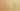# RightToLeftChaining

Misconception:

When chaining methods or attributes, like with `a().b()`, `a.b()`, `a().b`, or `a.b`, members are accessed from right to left (first the right most member). Also when an object instantiation is involved, e.g., `C().a()`, the right member of a chaining operation does have precedence over the object instantiation (`C()`). Therefore statements such as `C().a()` are invalid: valid code is instead `(C()).a()`.Incorrect

Chained accesses are invoked from right to left

Correct

Chained accesses are invoked from left to right

## CorrectionHere is what's right.

The dot operator is left-associative: `a.b.c` is equivalent to `(a.b).c`. This implies that chained operations are evaluated from left to right.

The following two code snippets both invoke method `a()` and then invoke method `b()` on the object returned by method `a()`:

``````x = a()
x.b()``````
``a().b()``

Here we see a correct expression tree for the above expression (assuming that `a` returns an object of type `T`):

The correct way to execute chained method calls is from left to right (from the bottom to the top in the expression tree). For the example expression, first `a()` is called, then `b()` is called on the return value of `a()`. The fact that `b()` is called on the return value of `a()` implies that `a()` has to be called before `b()`, and thus that these calls evaluate from left to right.

This misconception applies to method calls (like `x.a().b()`), field accesses (like `x.f.g`), and any mix thereof (e.g., `o.a().f.b().h`).

## SymptomsHow do you know your students might have this misconception?

This misconception can manifest itself when drawing expression trees, e.g., the tree for `a().b()`, where students mistakenly construct the tree top-down (here, an `a()` node above a `b()` node).

Python